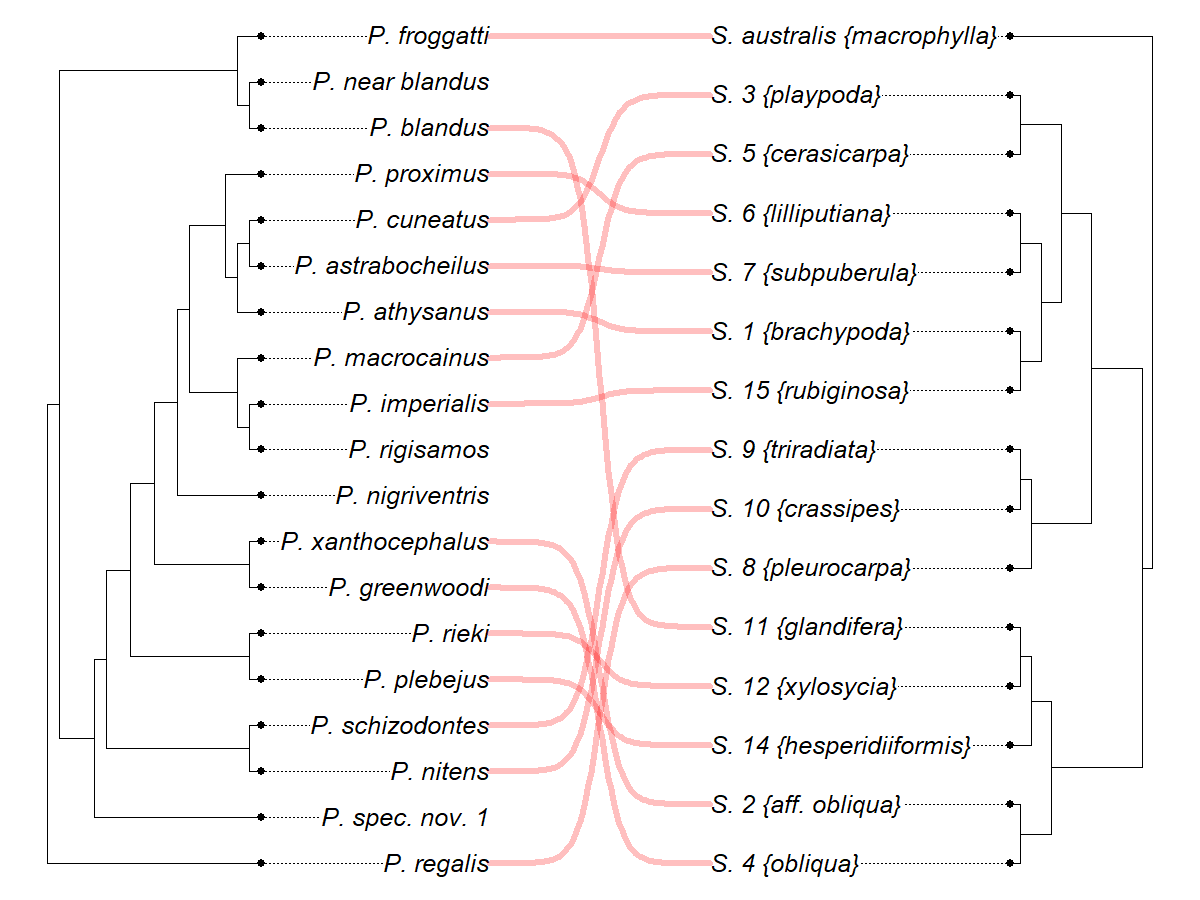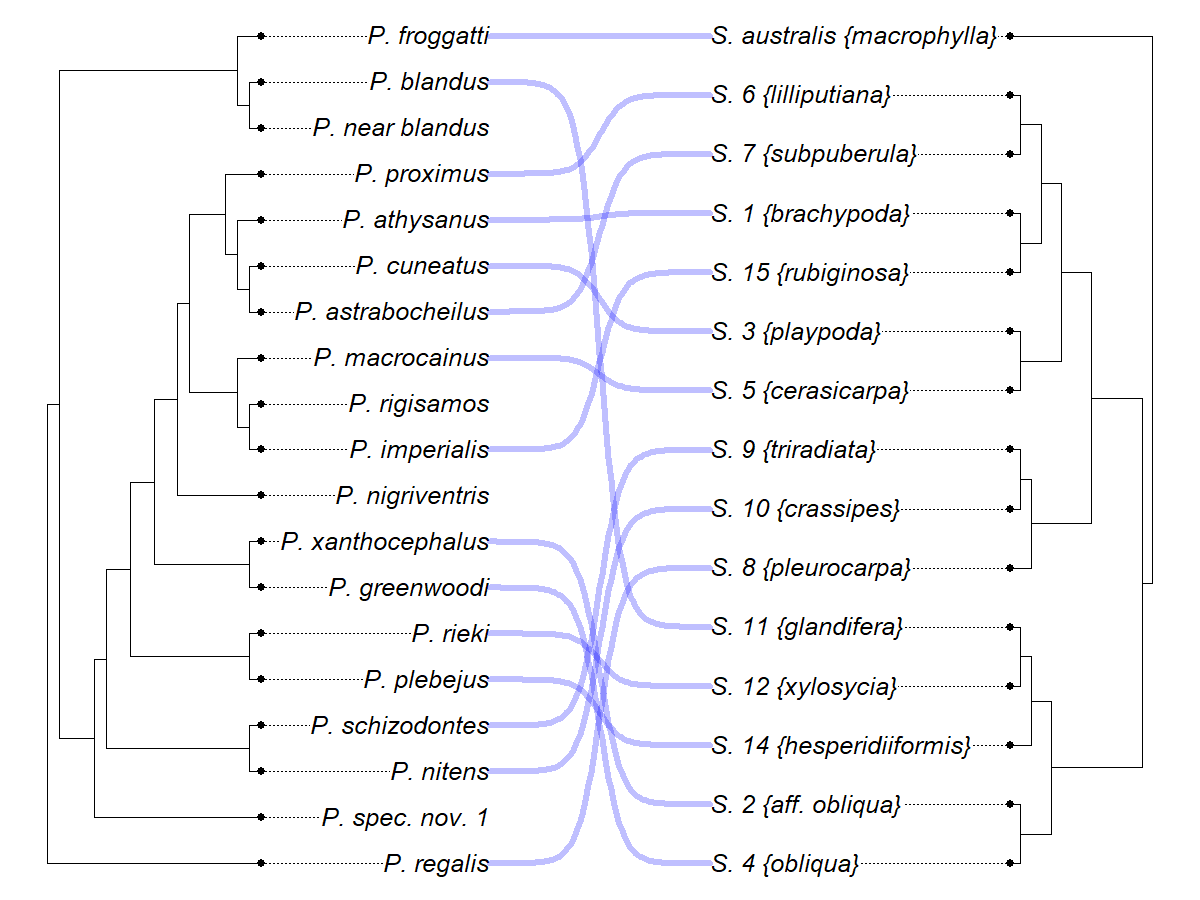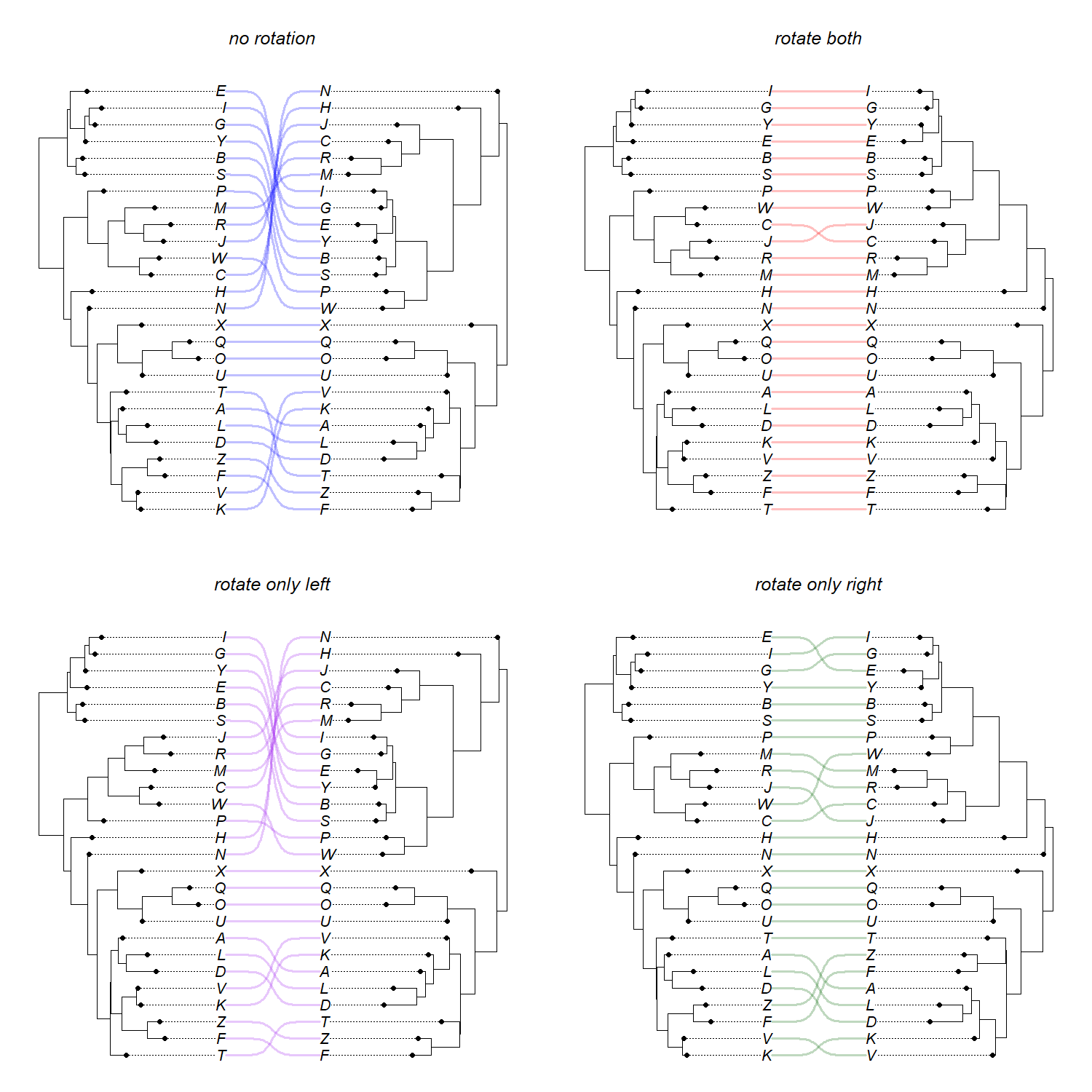## Sunday, June 5, 2022

### Co-phylogeny plots rotating only the left or the right trees to optimize alignment

This weekend I got the following message from a phytools user:

“Recently, I used the `cophylo` function of phytools for comparing two phylogenetic trees. In this particular analysis, ideally, I want to fix the topology of tree 1 while letting `cophylo` only rotate tree 2 to get rid of unnecessary crossing of the association lines. I did notice the `rotate=` parameter in `cophylo` but it seems to me that `rotate=TRUE` will rotate both trees, which is still different from what I want. So I was wondering if you can give me some suggestions.”

How to rotate one tree but not the other?

Well, this is a tiny bit tricky, but (of course!) possible.

So, let's say what we want to do is rotate only the second input tree (and not the first) when we create a `"cophylo"` object for plotting.

To demonstrate this, I'm going to load a tree and host-parasite association dataset from Lopez-Vaamonde et al. (2001).

``````library(phytools)
data(wasp.trees)
data(wasp.data)
``````

This is how it would normally work to create a `"cophylo"` object and plot it using these data (from the phytools `cophylo` help page).

``````## create co-phylogenetic object
wasp.cophylo<-cophylo(wasp.trees[],wasp.trees[],
assoc=wasp.data)
``````
``````## Rotating nodes to optimize matching...
## Done.
``````
``````## plot co-phylogenies
0.25))
``````Before we go any further, we should sidestep briefly and make sure that the tip labels of each of our input trees are in what ape calls “cladewise” order. We can do that using `phytools::untangle` as follows:

``````trees<-untangle(wasp.trees,"read.tree")
``````

Neat. Next, I'll also just rename our table of associations `assoc` to make our code a bit more easy to follow.

``````assoc<-wasp.data
``````

This table just gives the set of tips in tree 1 that are associated with tips in tree 2, or vice versa.

``````assoc
``````
``````##        Pleistodontes                Sycoscapter
## 1      P._greenwoodi             S._4_{obliqua}
## 2  P._xanthocephalus        S._2_{aff._obliqua}
## 3        P._plebejus   S._14_{hesperidiiformis}
## 4           P._rieki          S._12_{xylosycia}
## 5         P._blandus         S._11_{glandifera}
## 6         P._regalis         S._8_{pleurocarpa}
## 7          P._nitens          S._10_{crassipes}
## 9      P._imperialis         S._15_{rubiginosa}
## 10      P._athysanus          S._1_{brachypoda}
## 11 P._astrabocheilus         S._7_{subpuberula}
## 12       P._proximus        S._6_{lilliputiana}
## 13    P._macrocainus         S._5_{cerasicarpa}
## 14       P._cuneatus            S._3_{playpoda}
## 15      P._froggatti S._australis_{macrophylla}
``````

Now let's say we want to rotate only the second of the two trees.

Our first step will be to create a vector of the numerical order of the matches in our left tree to the taxa in our second, or right, tree.

``````yy<-setNames(sapply(assoc[,1],match,table=trees[]\$tip.label),
assoc[, 2])
yy
``````
``````##             S._4_{obliqua}        S._2_{aff._obliqua}   S._14_{hesperidiiformis}
##                          7                          8                          5
##          S._12_{xylosycia}         S._11_{glandifera}         S._8_{pleurocarpa}
##                          6                         18                          1
##                          3                          4                         10
##          S._1_{brachypoda}         S._7_{subpuberula}        S._6_{lilliputiana}
##                         15                         13                         16
##         S._5_{cerasicarpa}            S._3_{playpoda} S._australis_{macrophylla}
##                         12                         14                         19
``````

Next, we'll use the phytools function `tipRotate` (used internally by `cophylo`) to try to find the best rotation of the tips in our second tree with the tip order of the first tree. This is rotating only the second tree.

``````ROTATED<-tipRotate(trees[],
yy*Ntip(trees[])/Ntip(trees[]),
left=trees[],assoc=assoc,
method=c("pre","post"),print=TRUE)
``````
``````## objective: 224.113573407202
## objective: 224.113573407202
## objective: 224.113573407202
## objective: 224.113573407202
## objective: 224.113573407202
## objective: 224.113573407202
## objective: 224.113573407202
## objective: 224.113573407202
## objective: 224.113573407202
``````
``````## objective: 217.797783933518
## objective: 217.797783933518
## objective: 217.797783933518
## objective: 217.797783933518
## objective: 217.797783933518
``````

Finally let's create our `"cophylo"` object and plot it.

``````obj<-cophylo(trees[],ROTATED,assoc=assoc,
rotate=FALSE)
0.25))
``````These two different plot are not all that different – though they are different: only the right tree was rotated in the second plot.

We can also try to simulate an example where rotating only the left or the right trees (or both) will make more of a difference.

To that, I'll create a random starting tree (using `ape::rtree`) and then I'll randomly perturb it twice (using `phangorn::rNNI`). Then I'll create four `cophylo` plots: one without rotation; a second rotating both the left and the right trees; a third rotating only nodes in the left tree; and, finally, a fourth rotating nodes only in the right tree.

``````par(mfrow=c(2,2))
tree<-rtree(n=26,tip.label=LETTERS)
t1<-phangorn::rNNI(tree,10)
t2<-phangorn::rNNI(tree,10)
## rotate none
mar=c(1.1,1.1,4.1,1.1))
title(main="no rotation",font.main=3)
## rotate both
mar=c(1.1,1.1,4.1,1.1))
``````
``````## Rotating nodes to optimize matching...
## Done.
``````
``````title(main="rotate both",font.main=3)
## rotate only left or right
## rotate right only
assoc=data.frame(t1=t2\$tip.label,t2=t2\$tip.label)
yy<-setNames(sapply(assoc[,2],match,table=t2\$tip.label),
assoc[, 1])
ROTATED<-tipRotate(t1,yy*Ntip(t1)/Ntip(t2),
left=t1,assoc=assoc,method=c("pre","post"),
print=TRUE)
``````
``````## objective: 798
## objective: 798
## objective: 798
## objective: 798
## objective: 798
``````
``````## objective: 774
## objective: 774
``````
``````## objective: 726
## objective: 726
## objective: 726
## objective: 726
## objective: 726
## objective: 726
## objective: 726
## objective: 726
``````
``````## objective: 660
## objective: 660
``````
``````## objective: 640
``````
``````## objective: 632
``````
``````## objective: 628
## objective: 628
## objective: 628
``````
``````## objective: 624
## objective: 624
## objective: 624
``````
``````obj<-cophylo(ROTATED,t2,rotate=FALSE)
0.25),mar=c(1.1,1.1,4.1,1.1))
title(main="rotate only left",font.main=3)
## rotate right only
assoc=data.frame(t1=t1\$tip.label,t2=t1\$tip.label)
yy<-setNames(sapply(assoc[,1],match,table=t1\$tip.label),
assoc[, 2])
ROTATED<-tipRotate(t2,yy*Ntip(t2)/Ntip(t1),
left=t1,assoc=assoc,method=c("pre","post"),
print=TRUE)
``````
``````## objective: 798
``````
``````## objective: 798
## objective: 798
``````
``````## objective: 774
``````
``````## objective: 752
``````
``````## objective: 722
## objective: 722
## objective: 722
## objective: 722
## objective: 722
## objective: 722
## objective: 722
``````
``````## objective: 540
``````
``````## objective: 384
``````
``````## objective: 72
``````
``````## objective: 70
``````
``````## objective: 56
``````
``````## objective: 54
## objective: 54
## objective: 54
## objective: 54
## objective: 54
## objective: 54
## objective: 54
## objective: 54
``````
``````obj<-cophylo(t1,ROTATED,rotate=FALSE)